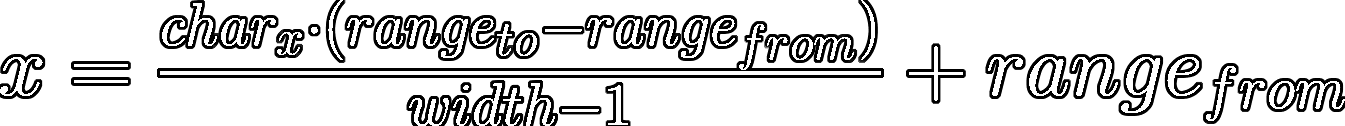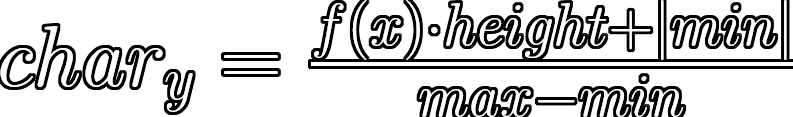Glyth Graph

Revision for 0.01

List of contents:

1. Brief Introduction
2. Process Overview
3. Technical Overview
4. Function Glossary
5. Code Example

Brief Introduction

Glyth Graph is an open-source python package, for graphing equations onto a 2-dimensional array (named the canvas) with a variety of arguments to draw within a specific range and bound. Scaling to the width and height of the canvas in proportion

.

Process Overview

glyth_graph_basic():

Upon attaching the constructor to an object a 2D array with the declared resolution size will be filled with the chosen blank_glyth, named the canvas.

draw_graph():

1. Check whether the given char_x (x-axis position of the canvas) is within the bounds of the canvas width as stated in the resolution.
2. If not formatted the equation will be simplified into an expression without ‘y’, ‘=’ and any spaces.
3. If not given the y-axis bounds for the equation within the x-axis range will be calculated by repetedly incrementing the x variable.
4. Then an x variable will be calculated by mapping the char_x from the width to the x-axis range of the equation, equally distributing each increment of char_x in the x-axis.
5. The x variable will be substitued into the equation to form a y-axis value, which will be mapped from the y-axis bounds of the equation to the canvas height.
6. Finally, the 2D coordinate of the char_x and char_y value on the canvas will be replaced by the chosen glyth.

Technical Overview

The package operates on mapping values between the x and f(x) from the graph equation to the given resolution of the canvas, translating coordinates with a non-uniform scaling factor to draw a glyth by a 2D index.

Notation form of the equation for mapping charx to xwhere rangefrom and rangeto are respectively the given x-axis region of the equation to draw.

Notation form of the equation for mapping f(x), equal to y, to charywhere max and min are respectively the calculated (or given) maximum and minimum y-axis values for the equation within the x-axis region.

Function Glossary

graph_basic(resolution: str, blank_glyth: str = None) -> None

The constructor of the class to create an attached object, setup the canvas array with the arguements given, both the size and blank (background) glyth

- resolution: the width by the height of the canvas measured in character glyths | 'x'.join([width, height])
- blank_glyth: the background glyth used for spacing the graph

format_equation(equation: str) -> str

Format the graph equation such that all unecessary characters are removed to be processed, this includes removal of 'y' and '=' if given an equation to form an expression and all ' ' (spaces) present

- equation: the mathematical equation of the graph going to be drawn

y_bounds(self, equation: str, x_range: tuple) -> tuple

Calculate the upper and lower bounds in the y-axis of a graph equation between the given x-axis range, to be used later for mapping positions

- equation: the mathematical equation of the graph going to be drawn
- x_range: a tuple of the x-axis range between which the graph will be used, all outside this is unnecessary

draw_graph(char_x: int, equation: str, glyth: str, x_range: tuple, y_bounds: tuple = None) -> list:

Draw a glyth onto the canvas array dependent on given arguments in relation to the graph equation, including the x-axis range and y-axis bounds of the 2-dimensional section of the graph and character position along the canvas

- char_x: the x_axis glyth position of the canvas, such that it starts to the leftmost position (0) to the rightmost (canvas width - 1) | 0 <= char_x < canvas width
- equation: the mathematical equation of the graph going to be drawn
- glyth: the character/s to be drawn onto the canvas at the calculated coordinate relative to the graph equation
- x_range: a tuple of the x-axis range between which the graph will be used, all outside this is unnecessary | (range_from, range_to)
- y_bounds: a tuple of the y-axis bounds for the x-axis region of the graph, including both the minimum and maximum values | (min, max)

clear_canvas() -> None:

Clear the canvas by replacing all indicies in the array with the blank glyth assigned in the constructor, removing any graphs drawn

print_canvas(clear: bool = None) -> None:

Pretty print the canvas array into equal rows of the set width with newline character moving to the next row, as each index is printed incrementally

- clear: a boolean value (either True or False) whether to clear the each canvas array index after printing the index | True or False

Code Example

A simple code example showing the usage of all functions in the package, with the user inputting variables to produce the wanted graph/s onto the canvas array as random Base64 character glyths:

from glyth_graph import graph_basic
from random import choice

character_set = '0123456789ABCDEFGHIJKLMNOPQRSTUVWXYZabcdefghijklmnopqrstuvwxyz+/'

print('---Glyth Graph---')

print('\n---Resolution---')
width = int(input('Width (chars): '))
height = int(input('Height (chars): '))

glyth_graph = graph_basic(
resolution = 'x'.join([str(width), str(height)]),
blank_glyth = '  '
)

while True:
print('\n---Graph Properties---')
equation = glyth_graph.format_equation(input('Equation: '))
range_from, range_to = int(input('x-axis From: ')), int(input('x-axis To: '))
print()

bounds = glyth_graph.y_bounds(
equation = equation,
x_range = (range_from, range_to)
)

for char_x in range(0, width):
glyth_graph.draw_graph(
char_x = char_x,
equation = equation,
glyth = choice(character_set),
x_range = (range_from, range_to),
y_bounds = bounds
)

glyth_graph.print_canvas()

An example of an output to the program, which can vary with custom values for all given inputs, pretty printing the canvas array:

---Glyth Graph---

---Resolution---
Width (chars): 100
Height (chars): 30

Width: 100 | Height: 30

---Graph Properties---
Equation: y = math.sin(x)
x-axis From: 0
x-axis To: 6.283185

LbvwLB+K
Rp8        49D
MB              FgW
Kt                   O
i6                      +w
t                          f
z                            LZ
k7                               q
9                                  q
Y                                    G
3                                      yP
r                                         c
9                                           h
C                                             4
f                                               K
l                                                 M                                               oe
o                                             7
y                                           n
O                                         e
tf                                      0
M                                    u
r                                  O
I                               lv
o8                            w
L                          A
Q2                      uO
w                   LD
zvu              8x
nGl        xMw
XsohPTDx

Permissions Conditions Limitations
Distribution Warranty
Modification
Private use

Copyright (c) 2021 Ivan (GitHub: ivanl-exe, E-Mail: [email protected])

Permission is hereby granted, free of charge, to any person obtaining a copy
of this software and associated documentation files (the "Software"), to deal
in the Software without restriction, including without limitation the rights
to use, copy, modify, merge, publish, distribute, sublicense, and/or sell
copies of the Software, and to permit persons to whom the Software is
furnished to do so, subject to the following conditions:

The above copyright notice and this permission notice shall be included in all
copies or substantial portions of the Software.

THE SOFTWARE IS PROVIDED "AS IS", WITHOUT WARRANTY OF ANY KIND, EXPRESS OR
IMPLIED, INCLUDING BUT NOT LIMITED TO THE WARRANTIES OF MERCHANTABILITY,
FITNESS FOR A PARTICULAR PURPOSE AND NONINFRINGEMENT. IN NO EVENT SHALL THE
AUTHORS OR COPYRIGHT HOLDERS BE LIABLE FOR ANY CLAIM, DAMAGES OR OTHER
LIABILITY, WHETHER IN AN ACTION OF CONTRACT, TORT OR OTHERWISE, ARISING FROM,
OUT OF OR IN CONNECTION WITH THE SOFTWARE OR THE USE OR OTHER DEALINGS IN THE
SOFTWARE.

View Github